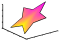# fill3

Filled 3-D polygons## Syntax

```fill3(X,Y,Z,C) fill3(X,Y,Z,ColorSpec) fill3(X1,Y1,Z1,C1,X2,Y2,Z2,C2,...) fill3(...,'PropertyName',PropertyValue) fill3(ax,...) h = fill3(...) ```

## Description

The `fill3` function creates flat-shaded and Gouraud-shaded polygons.

`fill3(X,Y,Z,C)` fills three-dimensional polygons. `X`, `Y`, and `Z` triplets specify the polygon vertices. If `X`, `Y`, or `Z` is a matrix, `fill3` creates n polygons, where n is the number of columns in the matrix. `fill3` closes the polygons by connecting the last vertex to the first when necessary. The values in `X`, `Y`, and `Z` can be numeric, datetime, duration, or categorical values.

`C` specifies color, where `C` is a vector or matrix of indices into the current colormap. If `C` is a row vector, `length(C)` must equal `size(X,2)` and `size(Y,2)`; if `C` is a column vector, `length(C)` must equal `size(X,1)` and `size(Y,1)`.

`fill3(X,Y,Z,ColorSpec)` fills the polygons with the color specified by `ColorSpec`, which can be one of the following values:

• A color name or a short name: `'red'` (or `'r'`), `'green'` (or `'g'`), `'blue'` (or `'b'`), `'cyan'` (or `'c'`), `'magenta'` (or `'m'`), `'yellow'` (or `'y'`), `'black'` (or `'k'`), `'white'` (or `'w'`).

• An RGB triplet specified as a 1-by-3 vector whose elements are the intensities of the red, green, and blue components of a color. The intensities must be in the range [0, 1]. For example, `[0.7 0.8 1]` specifies light blue.

`fill3(X1,Y1,Z1,C1,X2,Y2,Z2,C2,...)` specifies multiple filled three-dimensional areas.

`fill3(...,'PropertyName',PropertyValue)` allows you to set values for specific patch properties.

`fill3(ax,...)` creates the polygons in the axes specified by `ax` instead of in the current axes (`gca`). The option `ax` can precede any of the input argument combinations in the previous syntaxes.

`h = fill3(...)` returns a vector of patch objects.

## Examples

collapse all

Create four triangles with interpolated colors.

```X = [0 1 1 2; 1 1 2 2; 0 0 1 1]; Y = [1 1 1 1; 1 0 1 0; 0 0 0 0]; Z = [1 1 1 1; 1 0 1 0; 0 0 0 0]; C = [0.5000 1.0000 1.0000 0.5000; 1.0000 0.5000 0.5000 0.1667; 0.3330 0.3330 0.5000 0.5000]; figure fill3(X,Y,Z,C)```## Algorithms

If `X`, `Y`, and `Z` are matrices of the same size, `fill3` forms a vertex from the corresponding elements of `X`, `Y`, and `Z` (all from the same matrix location), and creates one polygon from the data in each column.

If `X`, `Y`, or `Z` is a matrix, `fill3` replicates any column vector argument to produce matrices of the required size.

If you specify color using the `ColorSpec` argument, `fill3` generates flat-shaded polygons and sets the patch object `FaceColor` property to an RGB triplet.

If you specify color using `C`, `fill3` scales the elements of `CLim` by the axes property `CLim`, which specifies the color axis scaling parameters, before indexing the current colormap.

If `C` is a row vector, `fill3` generates flat-shaded polygons and sets the `FaceColor` property of the patch objects to `'flat'`. Each element becomes the `CData` property value for the respective patch object.

If `C` is a column vector or a matrix, `fill3` generates polygons with interpolated colors and sets the patch object `FaceColor` property to `'interp'`. `fill3` uses a linear interpolation of the vertex colormap indices when generating polygons with interpolated colors. The elements in one column become the `CData` property value for the respective patch object. If `C` is a column vector, `fill3` replicates the column vector to produce the required sized matrix.

## Compatibility Considerations

expand all

Behavior changed in R2021a

## Extended Capabilities

### Topics

Introduced before R2006a